Courses
Courses for Kids
Free study material
Free LIVE classes
More

# Protractor Definition in Geometry for KidsLIVE
Join Vedantu’s FREE Mastercalss

## An Introduction to Protractor

A protractor is a transparent plastic device used for measuring angles. The simplest protector includes a semicircle disc ranging in degrees from 0⁰ to 180⁰. The first real protectors were made 500 years ago. By the 17th century, protractors were used by sailors for navigation at sea. Later, by the 18th century, protractors were widely used in Geometry and Mathematics.

## Definition of Protractor

Protractor is defined as a device used for measuring angles. The protectors we find in geometry boxes are generally made up of plastic, but there are other protractors that are made up of wood, steel, and also in glasses. Often, protractors measure angles in degrees but there are few protractors that measure angles in radians. These are termed as Radian-Scale Protractor.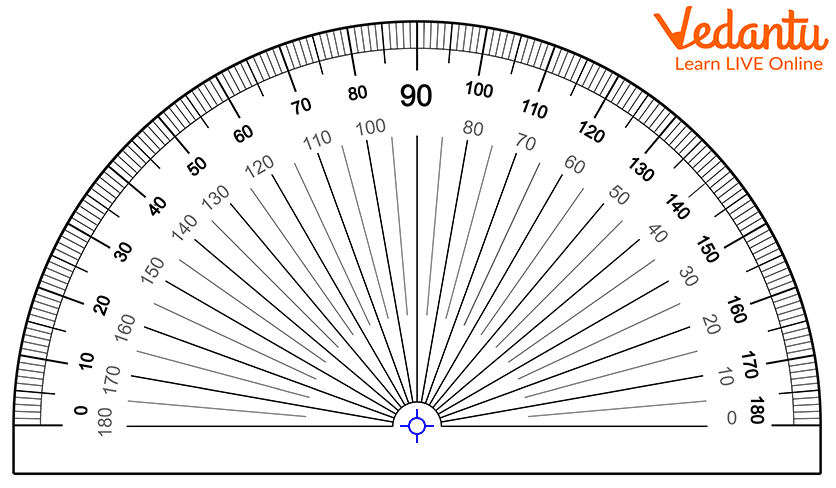Protractor

## Protractor Shapes

Protractors are available in two shapes. The two shapes of the protractor are:

• Semi-circular Shape

• Circular Shape

The semi circle shaped protractor is split into 180 equal parts which shows angle measurement from 0⁰ to 180⁰ as shown in the image given below.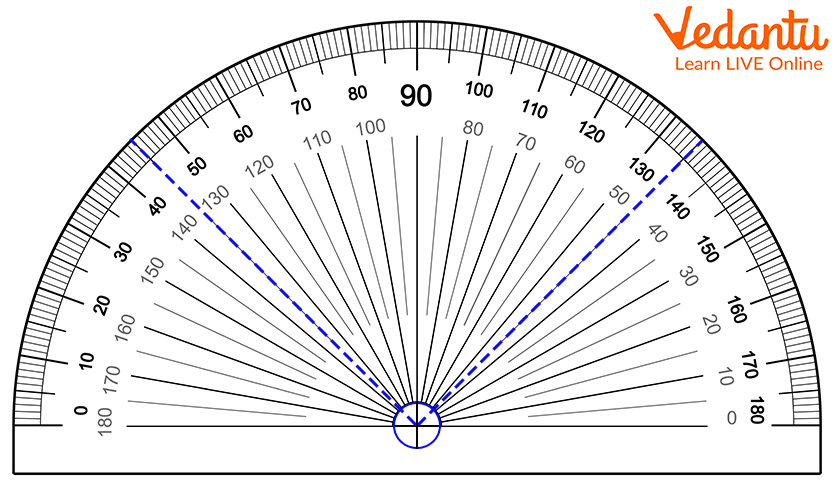Semi-circular shaped protractor

Similarly, the circular shaped protractor given below shows angle measurement from 0⁰ to 360⁰ as shown in the figure below.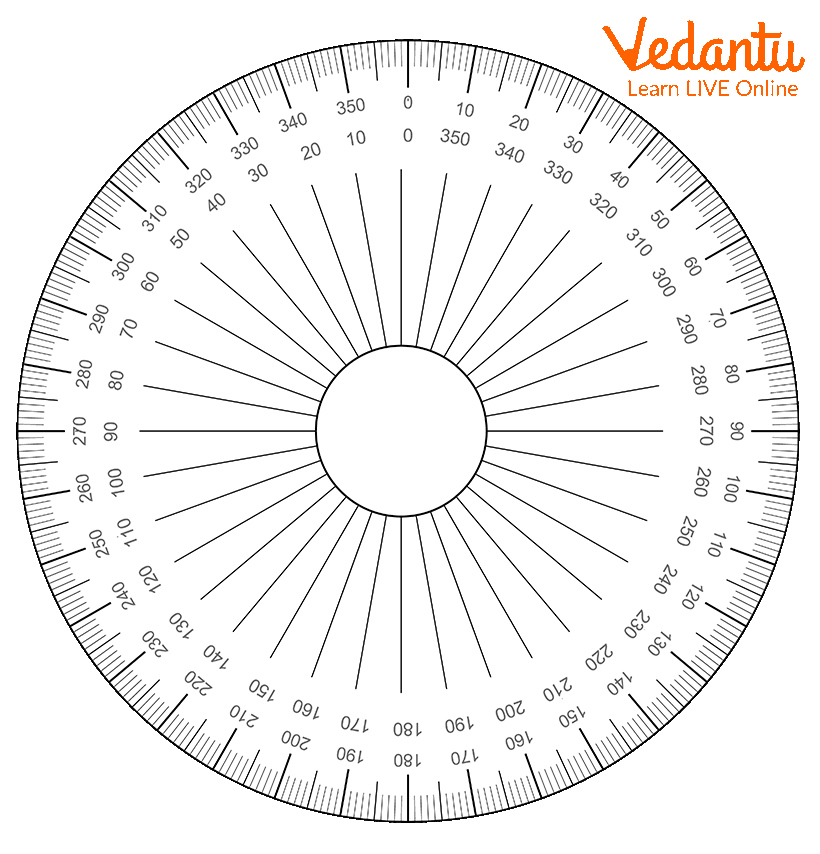Circular shape protractor

## Scales of Protractor

There are two scales of protractor. The two scales are:

• Outer Scales: The outer scale runs from 0 to 180 degrees going clockwise.

• Inner Scale: The inner scale runs from 0 to 180 degrees going anticlockwise.

Note: If angle opens to the right sides from the centre of the protractor, use the inner scale. On the other hand, If the angel opens to the left side from the centre of the protractor, use the outer scale.

Also remember, while constructing the acute angles (i.e. angles less than 90 degrees), also mark the angles on the right side of the protractor.

Contrarily, while constructing the obtuse angles (i.e.angles more than 90 degrees but less than 180 degrees), always mark the angles on the left side from the centre of the protractor.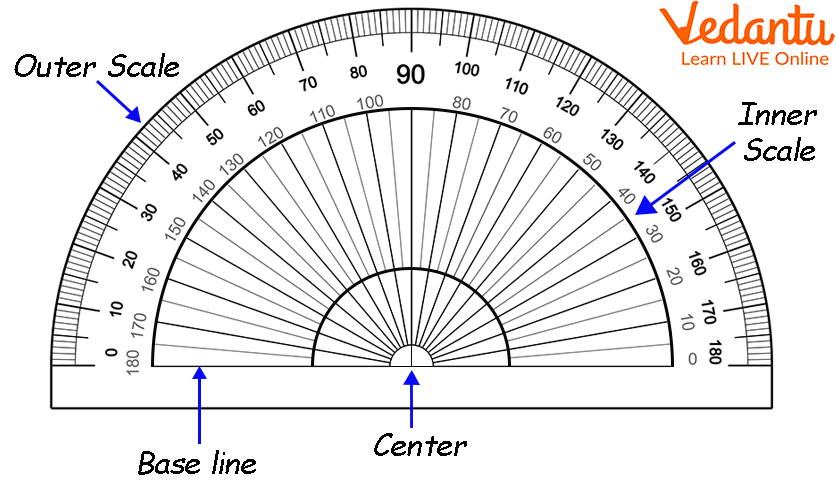Inner and outer scales of the protractor

Let’s learn how to construct and measure an angle using a protractor.

## How to Construct an Angle Using the Protractor?

Let's construct an angle whose measure is 60 degrees. Here, we will construct ∠XYZ= 60°.

Material Required: Pencil, Protractor, and Ruler.

Following are the steps to construct ∠XYZ= 60°.

Step 1: Draw a line YZ using a ruler.

Step 2: Place your protractor over the line YZ such that the baseline of the protractor co-exist with the line YZ and the midpoint or the centre of the baseline of the protractor co-exist with the point Y.

Step 3: Mark a point X where you find 60 degrees on the right side from the centre of the protractor as 60 degrees is an acute angle.

Step 4: Join XY using the ruler.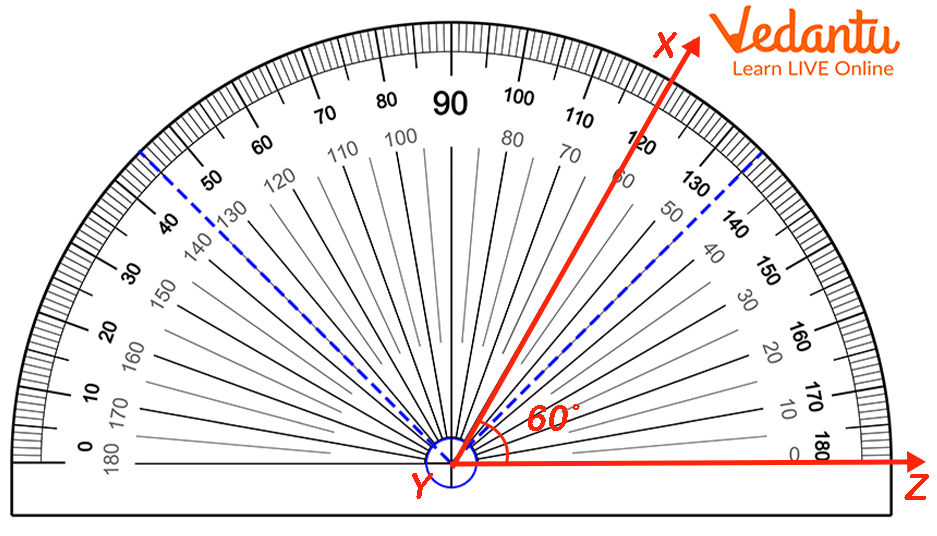Construction-of--∠60⁰-using-protractor

## How to Measure Angles Using a Protractor?

To measure an angle ABC using a protractor, place the midpoint or centre of the protractor on the vertex O of the angle. The base OA arm is placed on the baseline of the protractor as shown in the figure given below.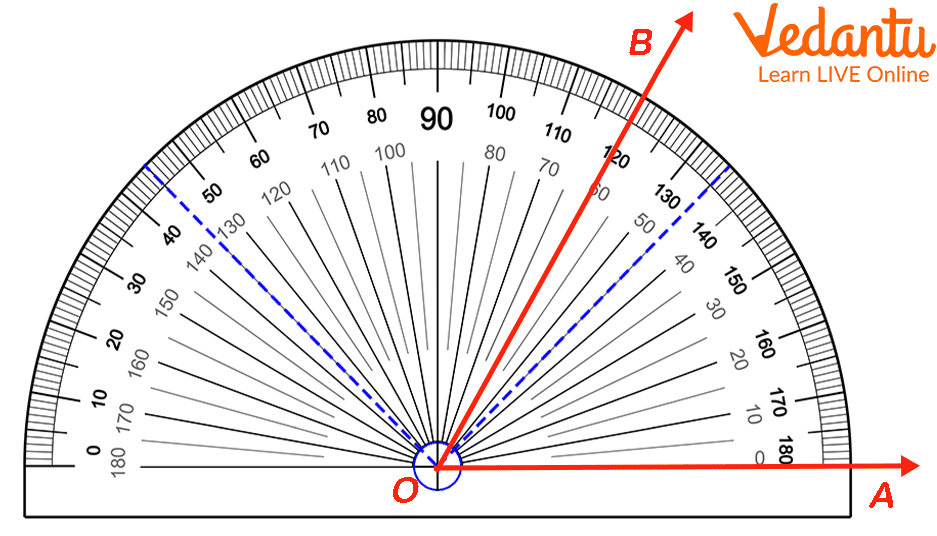∠AOB = 60°

Now, measure the angle on the protractor counting from 0 up to the point where the arm OB lies on the protractor. As the angle in the above figure is opened on the right side from the centre of the protractor, we will use the inner scale. Accordingly, ∠AOB = 60°.

## Conclusion

In short, a protractor is a measuring device, generally made up of transparent plastic or glass, used for drawing angles. The most commonly found protractor is semi-circular in shape ranging from 0° to 180°. Apart from drawing angles using protractors, we can also draw angles using a compass and measure those angles using protractors by applying certain geometrical properties.

Last updated date: 25th Sep 2023
Total views: 132.3k
Views today: 2.32k

## FAQs on Protractor Definition in Geometry for Kids

1. What is known as the baseline and origin of the protractor?

A horizontal straight line shown at the bottom of the protractor is known as the baseline of the protractor. The baseline is also referred to as the base of the protractor.

A point where the baseline line and the perpendicular line (also known as the 90-degree line) intersect at the bottom of the protractor is known as the origin or center of the protractor.

2. What defines a 360-degree protractor?

A 360-degree protractor is circular in shape and has reading ranges from 0° to 360° in the clockwise direction. The 360° protractor is commonly used in the field of architecture, mechanical drawings, and metrology.